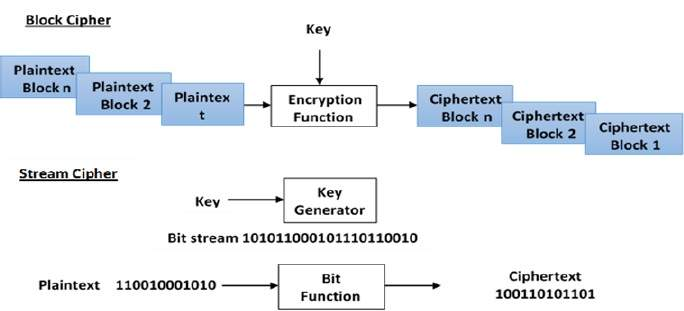# Modern Symmetric Key Encryption

Digital data is represented in strings of binary digits (bits) unlike alphabets. Modern cryptosystems need to process this binary strings to convert in to another binary string. Based on how these binary strings are processed, a symmetric encryption schemes can be classified in to −

## Block Ciphers

In this scheme, the plain binary text is processed in blocks (groups) of bits at a time; i.e. a block of plaintext bits is selected, a series of operations is performed on this block to generate a block of ciphertext bits. The number of bits in a block is fixed. For example, the schemes DES and AES have block sizes of 64 and 128, respectively.

## Stream Ciphers

In this scheme, the plaintext is processed one bit at a time i.e. one bit of plaintext is taken, and a series of operations is performed on it to generate one bit of ciphertext. Technically, stream ciphers are block ciphers with a block size of one bit.